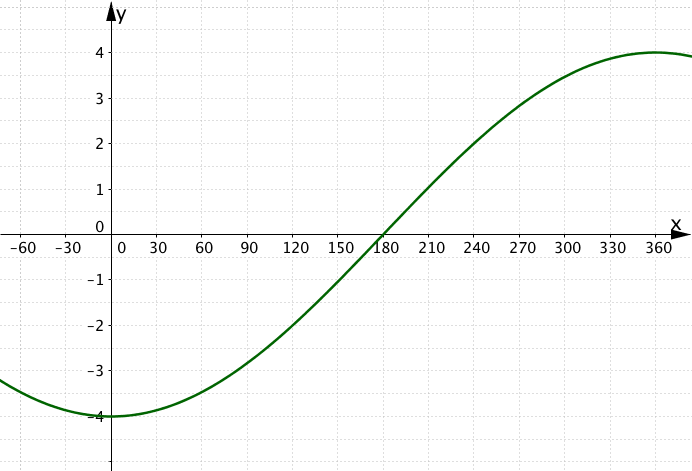# Sine and Cosine Transformations

When identifying a sinusoidal function, you may use the basic shape of either the sine curve or the cosine curve – one is just a horizontal transformation of the other!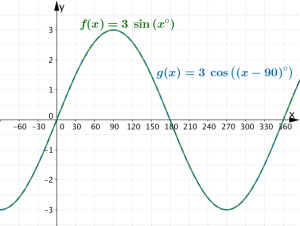The curve in the figure above can take the equationor.

Use the applet to view the four basic shapes.

## Exercise 1:

It has been noted above that. In other words, if you slide the cosine function 90 units to the right, your curve can be expressed as the sine function.

In a similar way, complete:

1.2.3.When a curve takes one of these four basic shapes shown in the applet, we can avoid using a horizontal transformation.

## Exercise 2:

Reproduce the following graphs on geogebra; using only functions of the formor(that is, no horizontal translation). Remember to use the degree symbol. A pc shortcut is ALT O; a mac short cut is SHIFT OPTION 8.

Graph 1: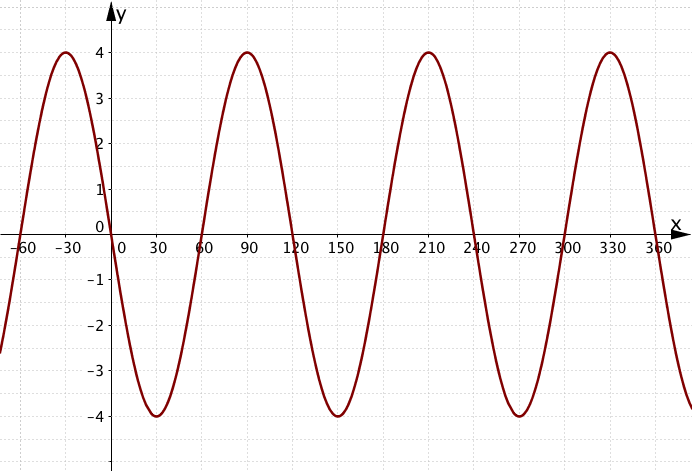Graph 2: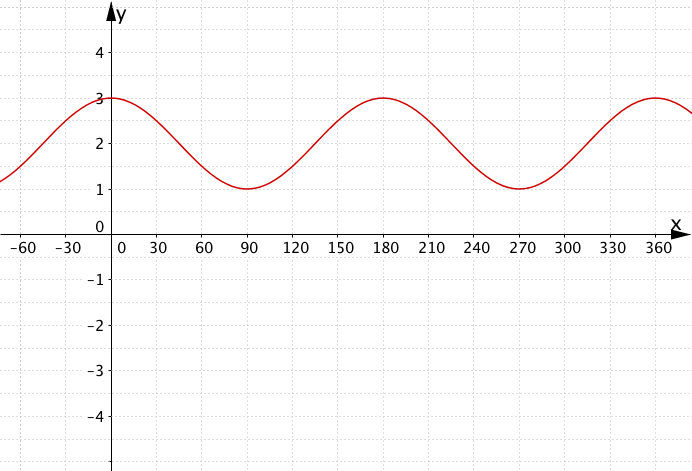Graph 3: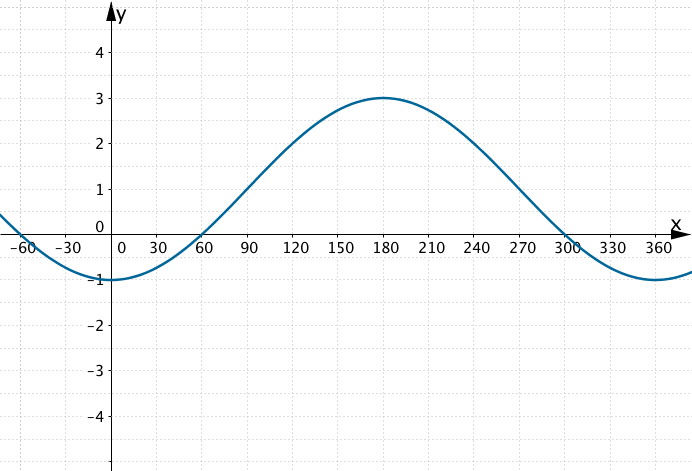Graph 4: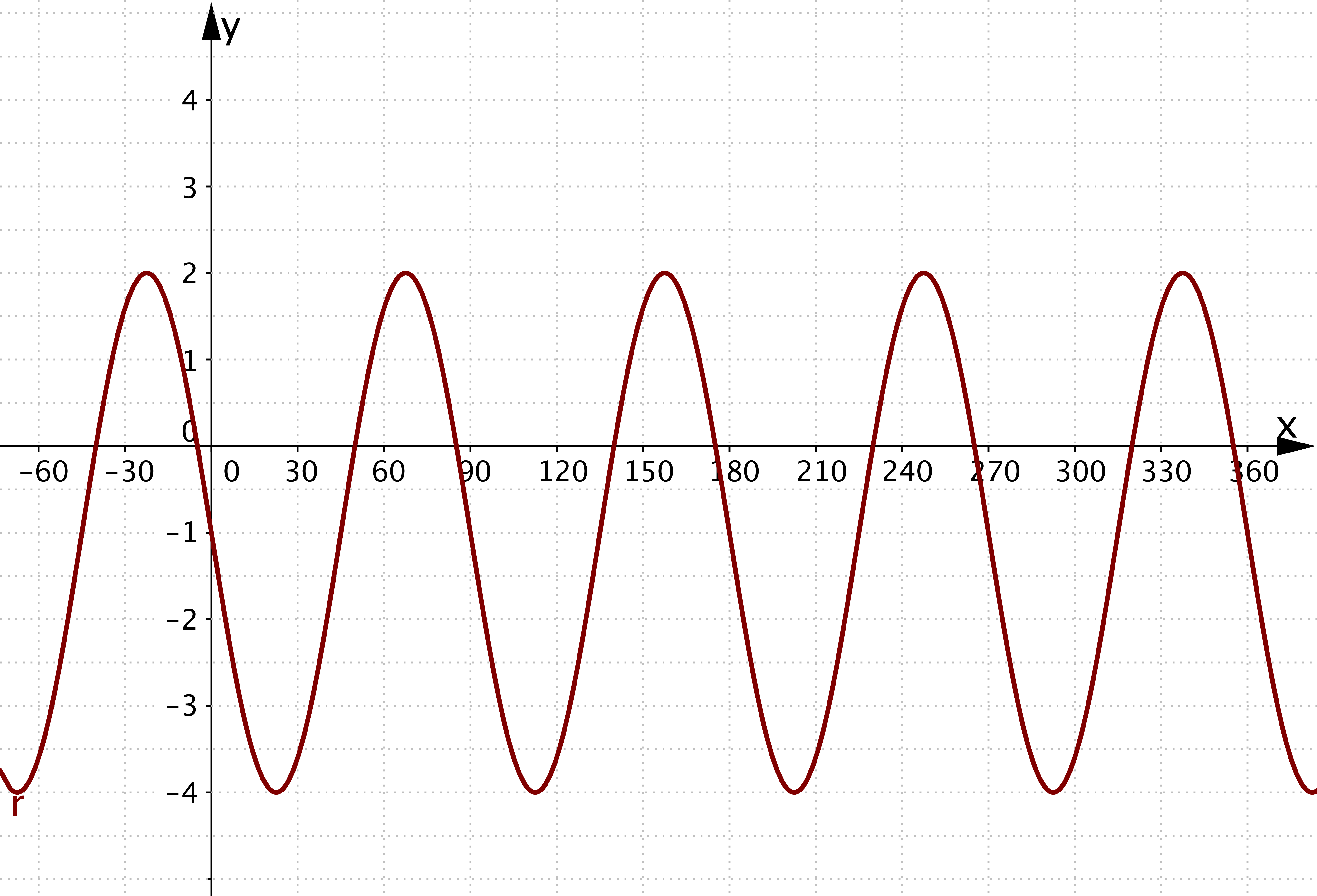Graph 5: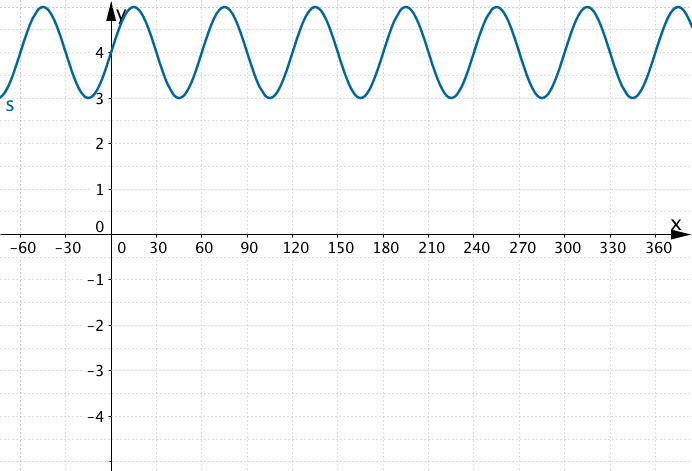Graph 6: